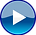top of page

# Introduction to Derivatives

## Basics of Derivative

Derivatives can be best understood by an example. Here is how easy it is to know what Derivatives are.

## Creation of Derivatives

While Derivatives may seem to be complex, it is easy to learn if the basics are right. Get to know the basics of derivatives.

## Building Blocks

It is important to understand some building blocks of Derivatives before getting into specifics.

## Derivatives - Basic Operation

While different derivatives operate differently, thefundamental operation of Derivatives is the same.

## Types & Primary Purpose of Derivative

This video throws light on the basic types of derivatives and the purpose of Derivatives

## Forwards

Understand how a Forward works and its formal definition

## Intro to Fx Derivatives

This video deals with the Forward contract as seen in Forex

## Need & Impact of Forward

Get to know the needs of a Forward contract and their impact

## Interest Rate Derivatives

Learn the basics of Interest rate derivatives

## Forward Rate Agreement

This video deals with the basics of Forward Rate Agreements and how FRA works

## Creation of Futures Contracts

Understand how Futures are created

## The Futures Contract

Look at how the Futures contract looks like and what are its features

## Swaps

A SWAP means an exchange! Get an introductory understanding of SWAPs

## Plain vanilla interest rate swap

Understand the manner in which a plain Vanilla Interest Rate Swap works

## Cross Currency Swap

Understanding Cross Currency SWAPs can be complex. Get to know the working of Cross Currency Swaps and its uses

## Credit Default Swap

Get to understand the basics of Credit default Swaps and how they workbottom of page